# RS Aggarwal Solutions for Class 7 Maths Exercise 20E Chapter 20 Mensuration

RS Aggarwal Solutions for Class 7 Maths Exercise 20E Chapter 20 Mensuration in simple PDF are given here. This exercise of RS Aggarwal Solutions for Class 7 Chapter 20 contains questions related to the circumference of a circle. The perimeter of a circle is called its circumference. The length of a thread that winds around the circle exactly gives the circumference of the circle. Students can practice RS Aggarwal Solutions for Class 7 Maths Chapter 20 Mensuration Exercise 20E with the help of solutions provided here.

## Download the PDF of RS Aggarwal Solutions For Class 7 Maths Chapter 20 Mensuration – Exercise 20E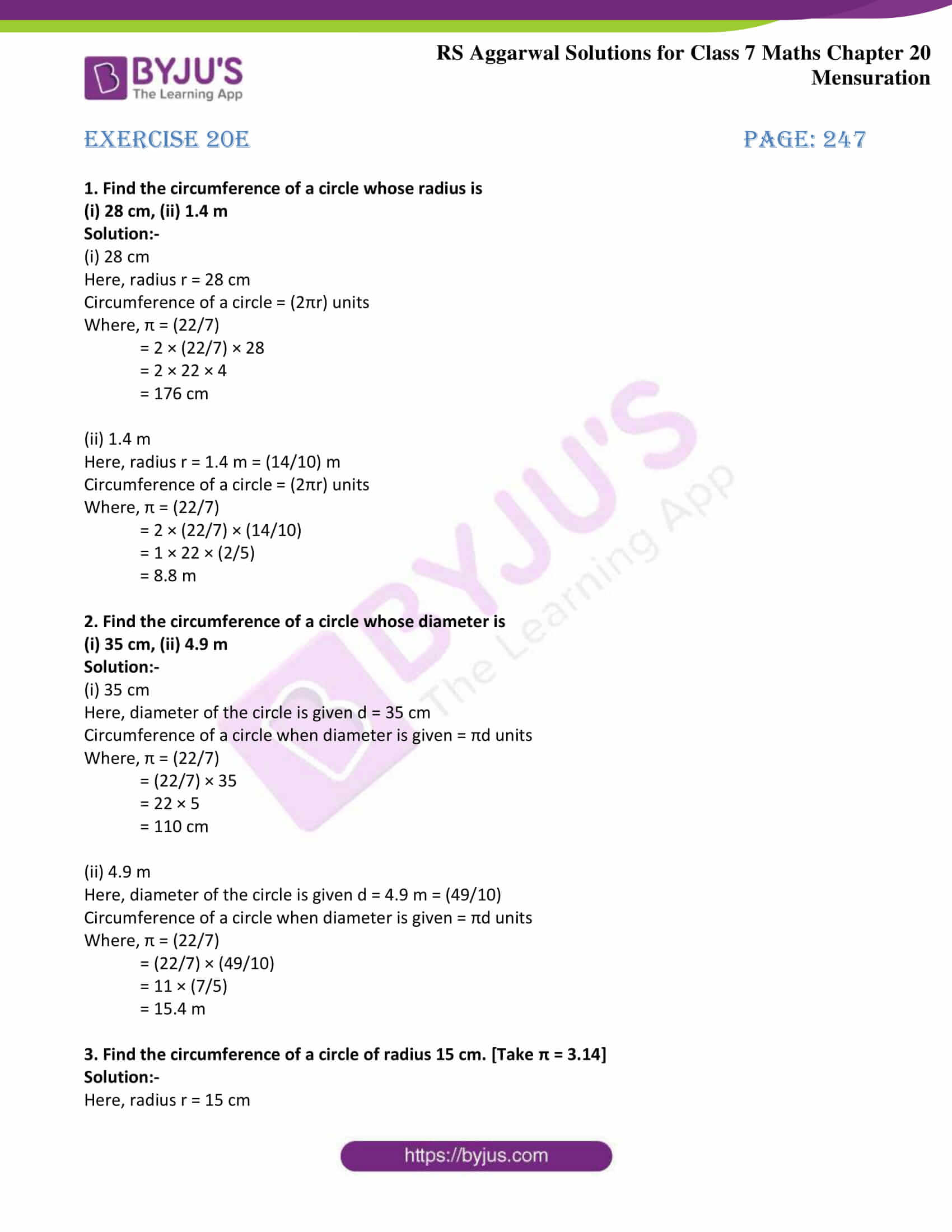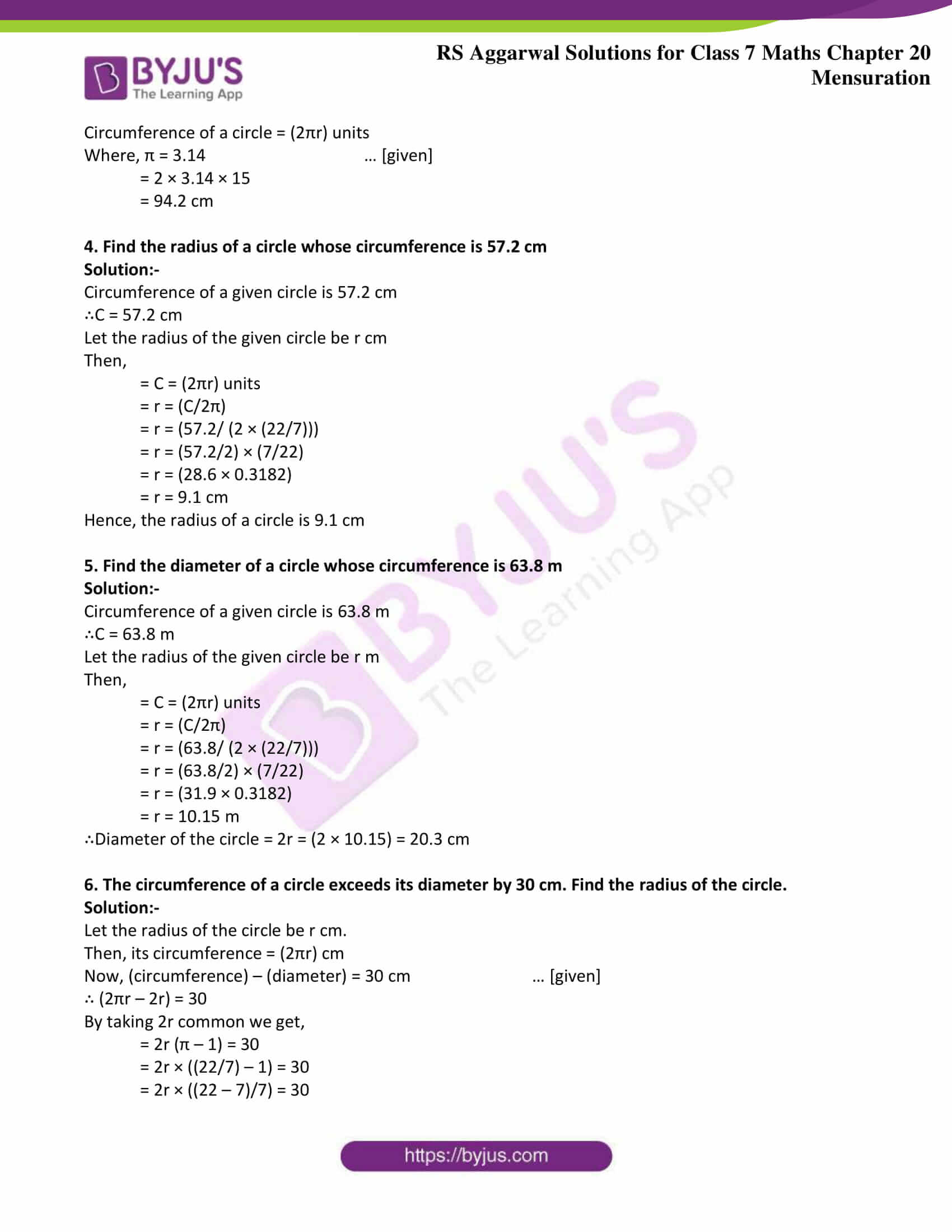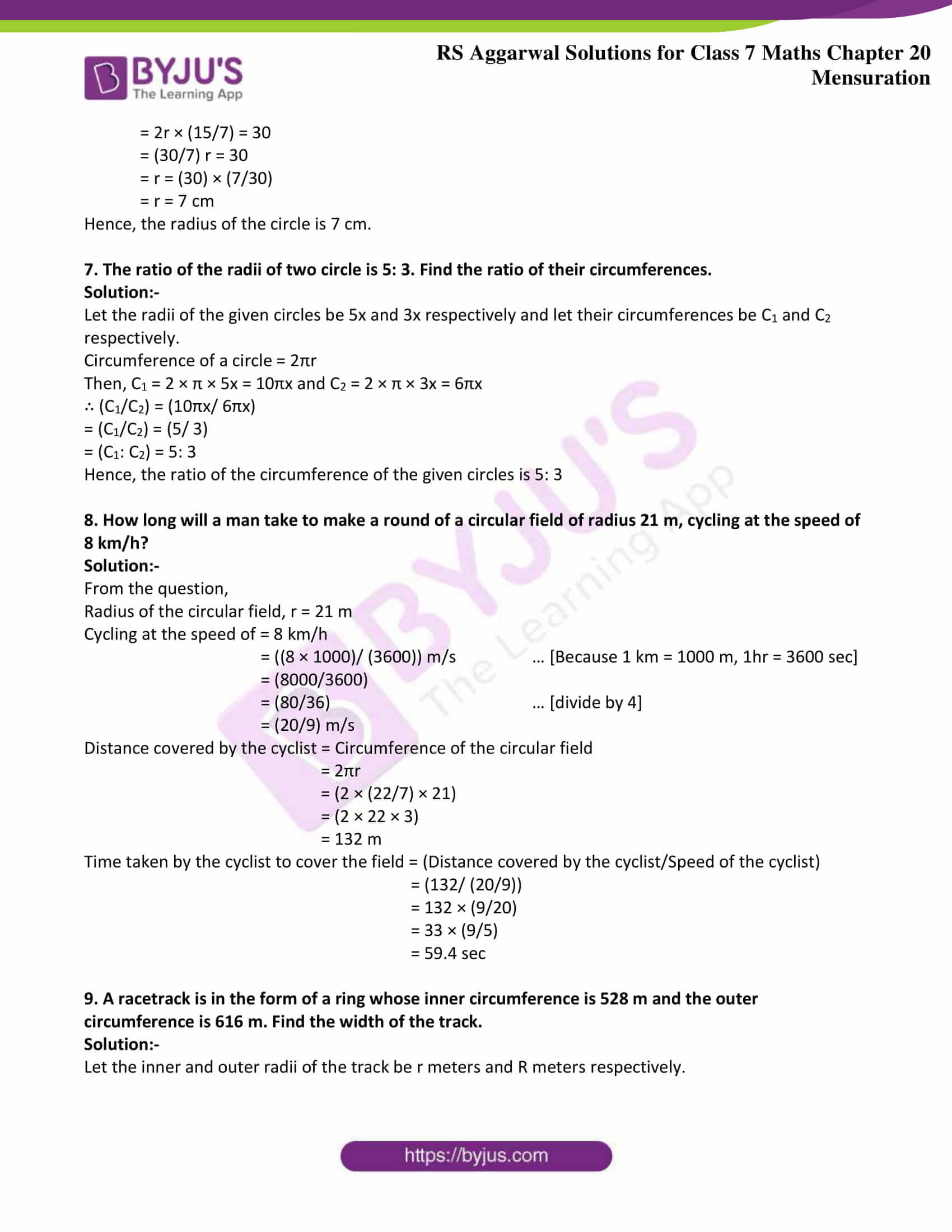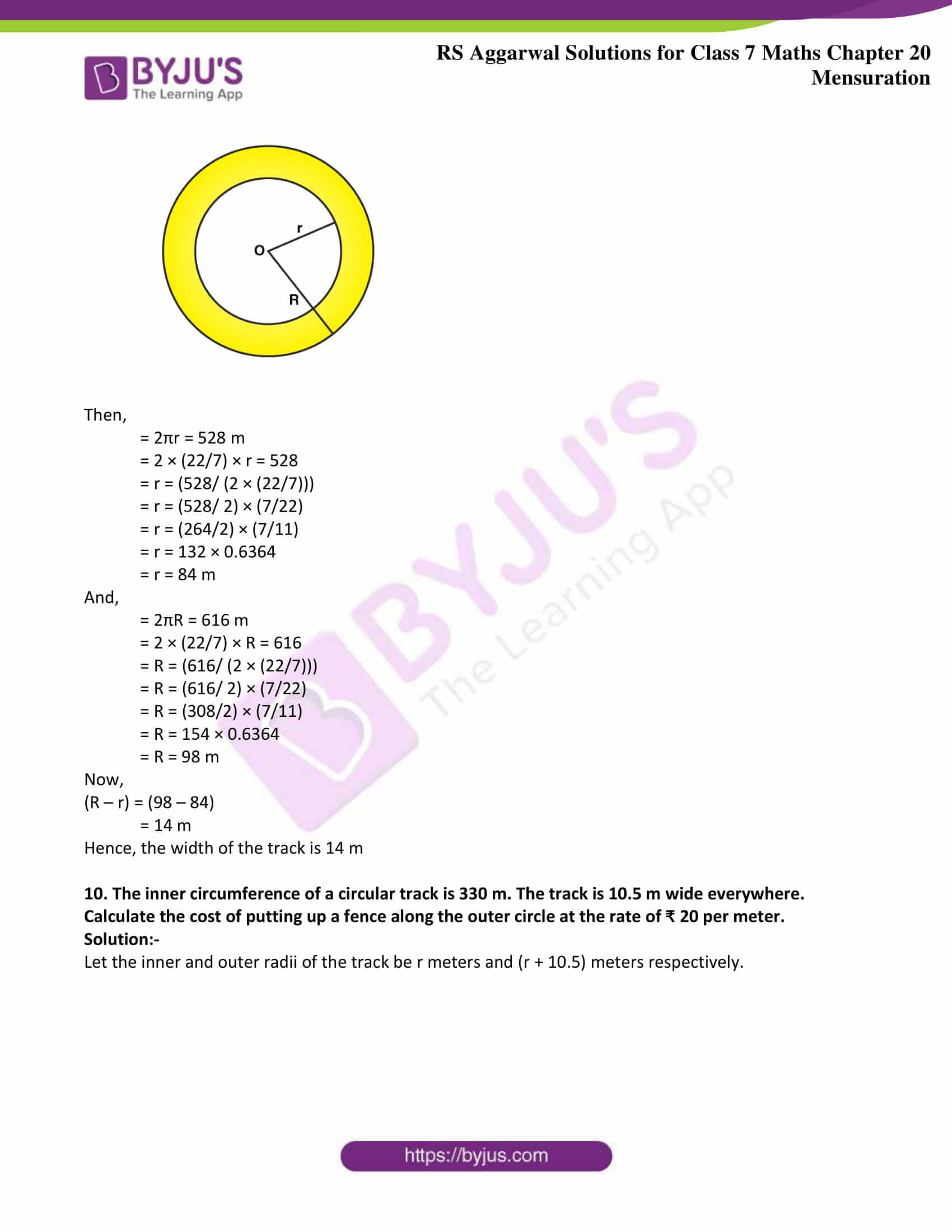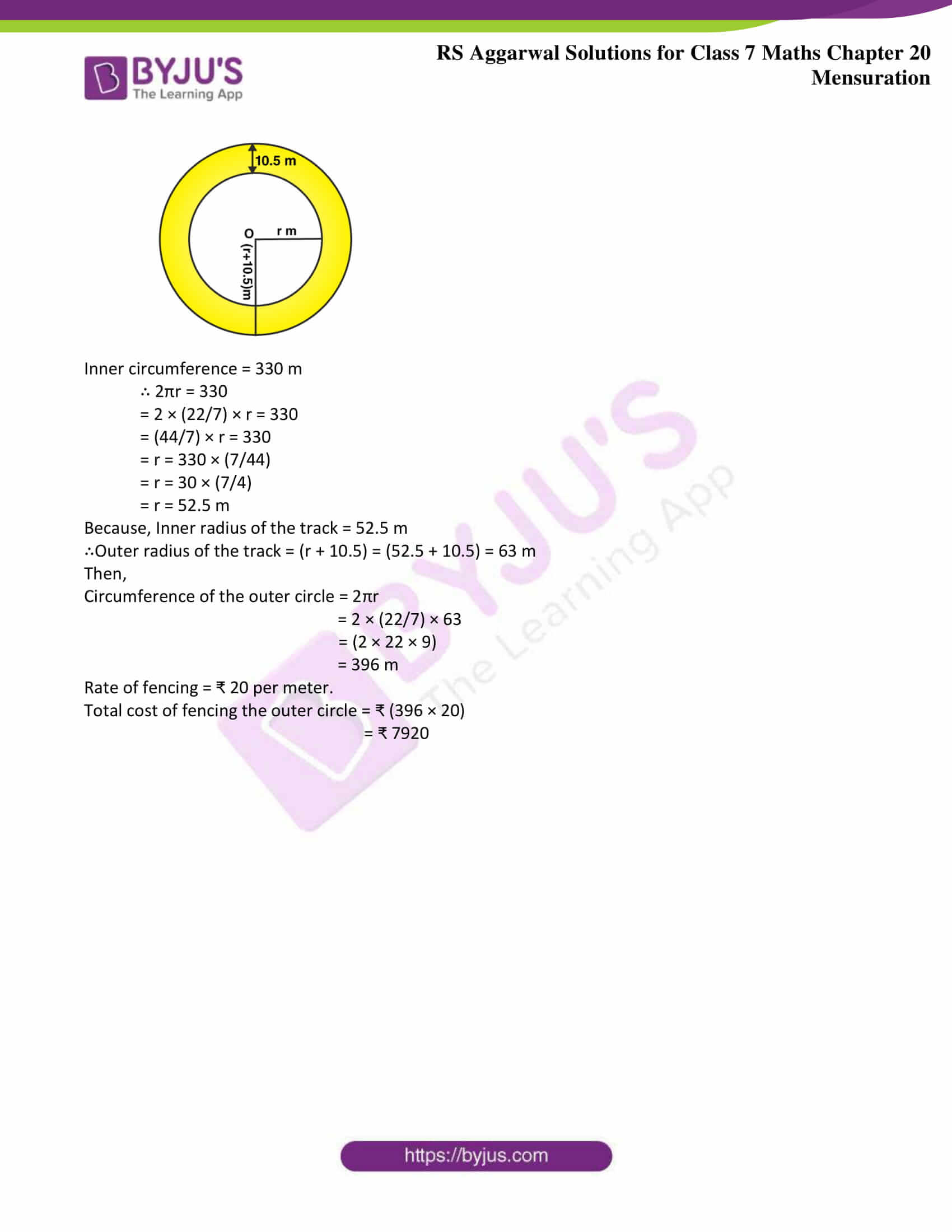### Access answers to Maths RS Aggarwal Solutions for Class 7 Chapter 20 – Mensuration Exercise 20E

1. Find the circumference of a circle whose radius is

(i) 28 cm, (ii) 1.4 m

Solution:-

(i) 28 cm

Here, radius r = 28 cm

Circumference of a circle = (2πr) units

Where, π = (22/7)

= 2 × (22/7) × 28

= 2 × 22 × 4

= 176 cm

(ii) 1.4 m

Here, radius r = 1.4 m = (14/10) m

Circumference of a circle = (2πr) units

Where, π = (22/7)

= 2 × (22/7) × (14/10)

= 1 × 22 × (2/5)

= 8.8 m

2. Find the circumference of a circle whose diameter is

(i) 35 cm, (ii) 4.9 m

Solution:-

(i) 35 cm

Here, diameter of the circle is given d = 35 cm

Circumference of a circle when diameter is given = πd units

Where, π = (22/7)

= (22/7) × 35

= 22 × 5

= 110 cm

(ii) 4.9 m

Here, diameter of the circle is given d = 4.9 m = (49/10)

Circumference of a circle when diameter is given = πd units

Where, π = (22/7)

= (22/7) × (49/10)

= 11 × (7/5)

= 15.4 m

3. Find the circumference of a circle of radius 15 cm. [Take π = 3.14]

Solution:-

Here, radius r = 15 cm

Circumference of a circle = (2πr) units

Where, π = 3.14 … [given]

= 2 × 3.14 × 15

= 94.2 cm

4. Find the radius of a circle whose circumference is 57.2 cm

Solution:-

Circumference of a given circle is 57.2 cm

∴C = 57.2 cm

Let the radius of the given circle be r cm

Then,

= C = (2πr) units

= r = (C/2π)

= r = (57.2/ (2 × (22/7)))

= r = (57.2/2) × (7/22)

= r = (28.6 × 0.3182)

= r = 9.1 cm

Hence, the radius of a circle is 9.1 cm

5. Find the diameter of a circle whose circumference is 63.8 m

Solution:-

Circumference of a given circle is 63.8 m

∴C = 63.8 m

Let the radius of the given circle be r m

Then,

= C = (2πr) units

= r = (C/2π)

= r = (63.8/ (2 × (22/7)))

= r = (63.8/2) × (7/22)

= r = (31.9 × 0.3182)

= r = 10.15 m

∴Diameter of the circle = 2r = (2 × 10.15) = 20.3 cm

6. The circumference of a circle exceeds its diameter by 30 cm. Find the radius of the circle.

Solution:-

Let the radius of the circle be r cm.

Then, its circumference = (2πr) cm

Now, (circumference) – (diameter) = 30 cm … [given]

∴ (2πr – 2r) = 30

By taking 2r common we get,

= 2r (π – 1) = 30

= 2r × ((22/7) – 1) = 30

= 2r × ((22 – 7)/7) = 30

= 2r × (15/7) = 30

= (30/7) r = 30

= r = (30) × (7/30)

= r = 7 cm

Hence, the radius of the circle is 7 cm.

7. The ratio of the radii of two circle is 5: 3. Find the ratio of their circumferences.

Solution:-

Let the radii of the given circles be 5x and 3x respectively and let their circumferences be C1 and C2 respectively.

Circumference of a circle = 2πr

Then, C1 = 2 × π × 5x = 10πx and C2 = 2 × π × 3x = 6πx

∴ (C1/C2) = (10πx/ 6πx)

= (C1/C2) = (5/ 3)

= (C1: C2) = 5: 3

Hence, the ratio of the circumference of the given circles is 5: 3

8. How long will a man take to make a round of a circular field of radius 21 m, cycling at the speed of 8 km/h?

Solution:-

From the question,

Radius of the circular field, r = 21 m

Cycling at the speed of = 8 km/h

= ((8 × 1000)/ (3600)) m/s … [Because 1 km = 1000 m, 1hr = 3600 sec]

= (8000/3600)

= (80/36) … [divide by 4]

= (20/9) m/s

Distance covered by the cyclist = Circumference of the circular field

= 2πr

= (2 × (22/7) × 21)

= (2 × 22 × 3)

= 132 m

Time taken by the cyclist to cover the field = (Distance covered by the cyclist/Speed of the cyclist)

= (132/ (20/9))

= 132 × (9/20)

= 33 × (9/5)

= 59.4 sec

9. A racetrack is in the form of a ring whose inner circumference is 528 m and the outer circumference is 616 m. Find the width of the track.

Solution:-

Let the inner and outer radii of the track be r meters and R meters respectively.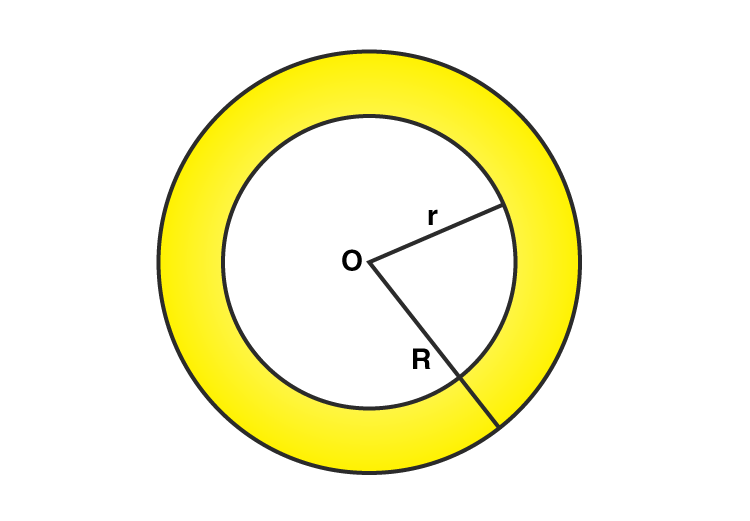Then,

= 2πr = 528 m

= 2 × (22/7) × r = 528

= r = (528/ (2 × (22/7)))

= r = (528/ 2) × (7/22)

= r = (264/2) × (7/11)

= r = 132 × 0.6364

= r = 84 m

And,

= 2πR = 616 m

= 2 × (22/7) × R = 616

= R = (616/ (2 × (22/7)))

= R = (616/ 2) × (7/22)

= R = (308/2) × (7/11)

= R = 154 × 0.6364

= R = 98 m

Now,

(R – r) = (98 – 84)

= 14 m

Hence, the width of the track is 14 m

10. The inner circumference of a circular track is 330 m. The track is 10.5 m wide everywhere. Calculate the cost of putting up a fence along the outer circle at the rate of ₹ 20 per meter.

Solution:-

Let the inner and outer radii of the track be r meters and (r + 10.5) meters respectively.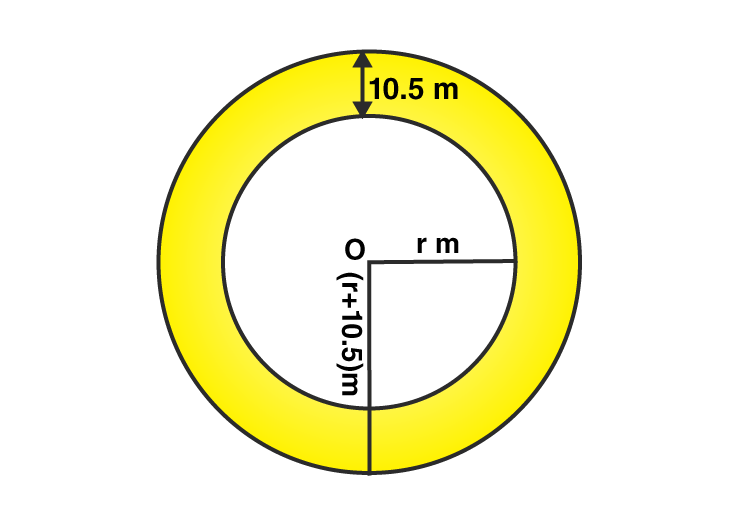Inner circumference = 330 m

∴ 2πr = 330

= 2 × (22/7) × r = 330

= (44/7) × r = 330

= r = 330 × (7/44)

= r = 30 × (7/4)

= r = 52.5 m

Because, Inner radius of the track = 52.5 m

∴Outer radius of the track = (r + 10.5) = (52.5 + 10.5) = 63 m

Then,

Circumference of the outer circle = 2πr

= 2 × (22/7) × 63

= (2 × 22 × 9)

= 396 m

Rate of fencing = ₹ 20 per meter.

Total cost of fencing the outer circle = ₹ (396 × 20)

= ₹ 7920

### Access other exercises of RS Aggarwal Solutions For Class 7 Chapter 20 – Mensuration

Exercise 20A Solutions

Exercise 20B Solutions

Exercise 20C Solutions

Exercise 20D Solutions

Exercise 20F Solutions

Exercise 20G Solutions